Function Repository Resource:

Generate three cyclic harmonic quadrangles from a reference triangle

Contributed by: Shenghui Yang (Wolfram Research)
 ResourceFunction["HarmonicQuadrilaterals"][{p1,p2,p3}] gives the three harmonic quadrilaterals corresponding to the triangle with corner points p1,p2, and p3.

## Details

A harmonic quadrilateral is a cyclic quadrangle in which the products of the lengths of opposite sides are equal.
Harmonic quadrilateral is closely related to pole/polar line, symmedian and other important concepts in projective and inversion geometry.
HarmonicQuadrilaterals effectively uses TriangleConstruct and reflects one vertex of the triangle with respect to the projection of the circumcenter on the symmedian cevian. This gurantees that the final polygon is always convex, given a proper ordering of the new point and that of the random triangle.
HarmonicQuadrilaterals gives a list of Polygon objects.

## Examples

### Basic Examples (3)

Find three harmonic quadrilaterals that contain a triangle:

 In:=Out=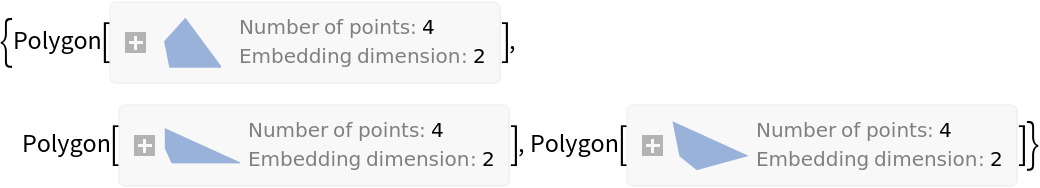Show these:

 In:=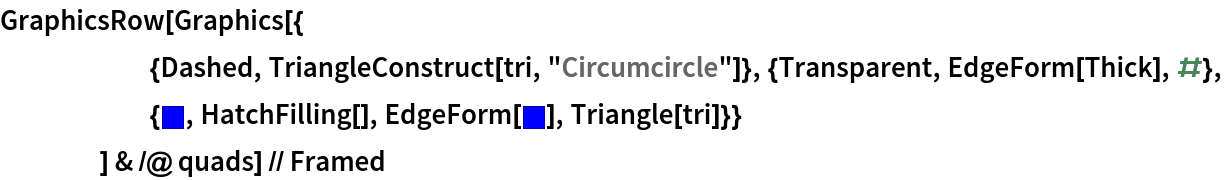Out=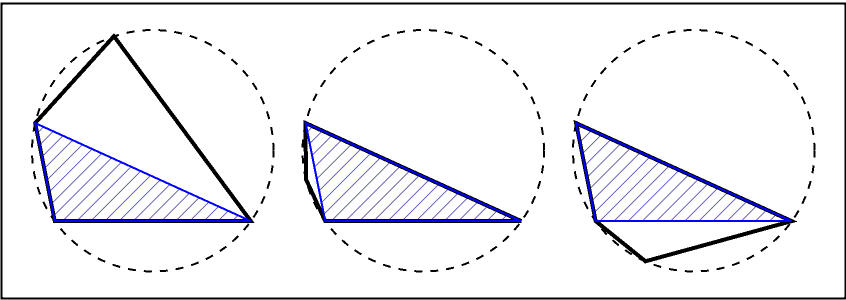The products of the lengths of opposite sides are equal:

 In:=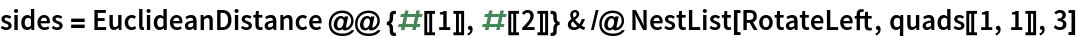Out=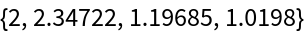In:=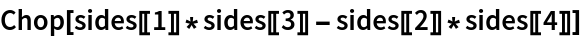Out=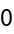### Scope (2)

Some spiral similar triangles pairs (marked as red and blue) can be found by halving the diagonal or the extension of a side:

 In:=Take the midpoint of one diagonal:

 In:=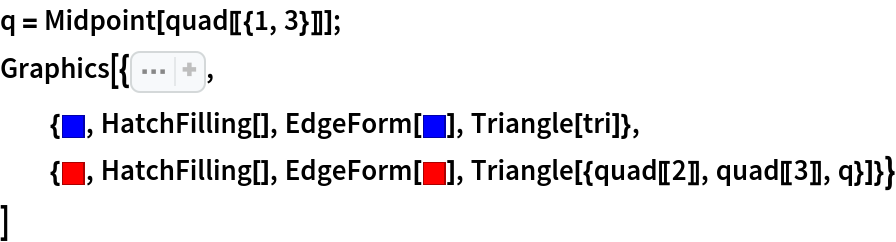Out=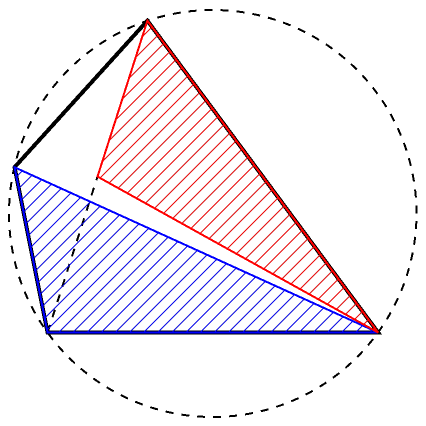In:=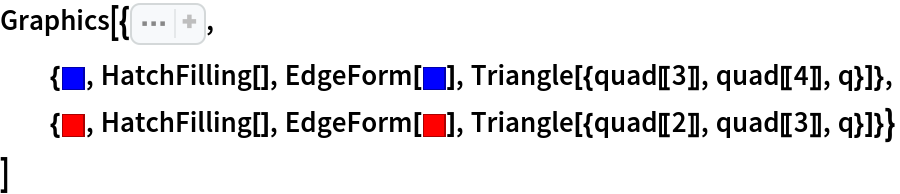Out=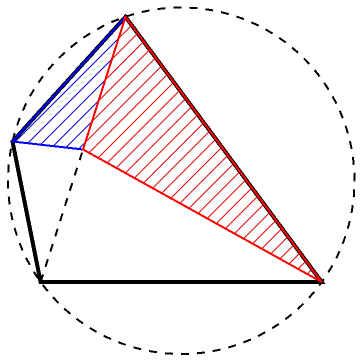In:=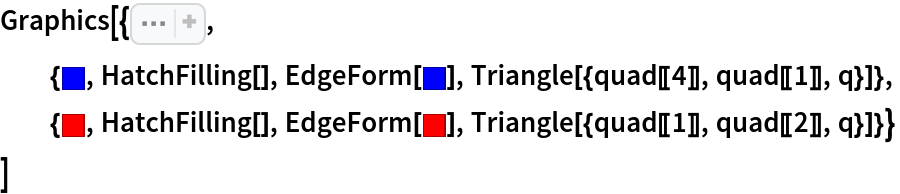Out=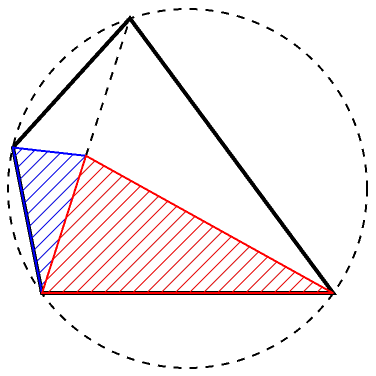Double the length of one side:

 In:=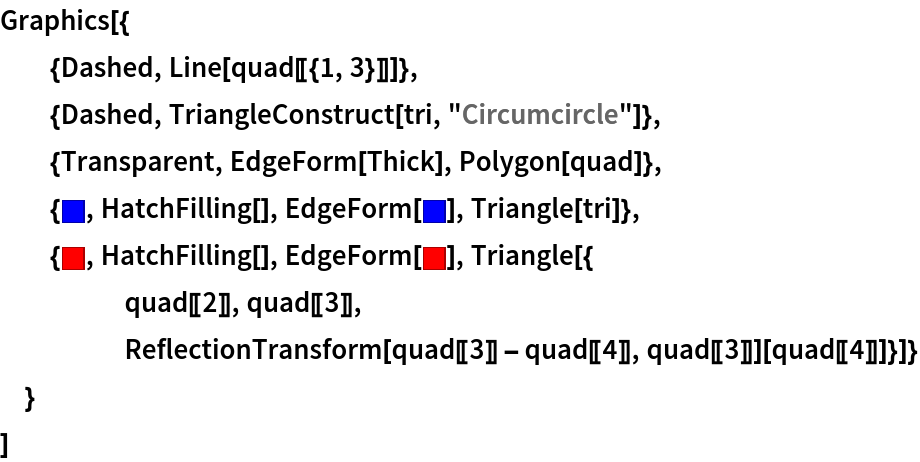Out=A harmonic quadrilateral can also be constructed with GeometricScene by equating the product of the lengths of opposite sides:

 In:=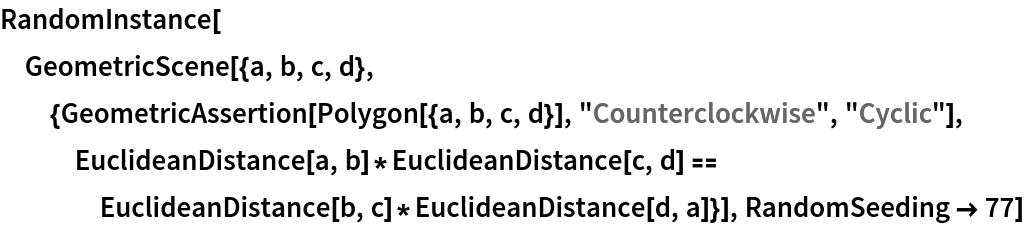Out=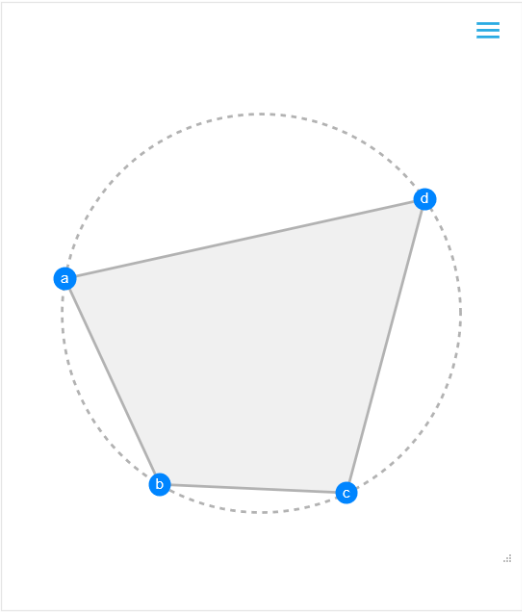### Neat Examples (3)

The vertices of a harmonic quadrilateral are inverse to some squares and vice versa. To find a proper inversion center, use the intersection of the orthogonal circles passing through the vertices of each diagonal:

 In:=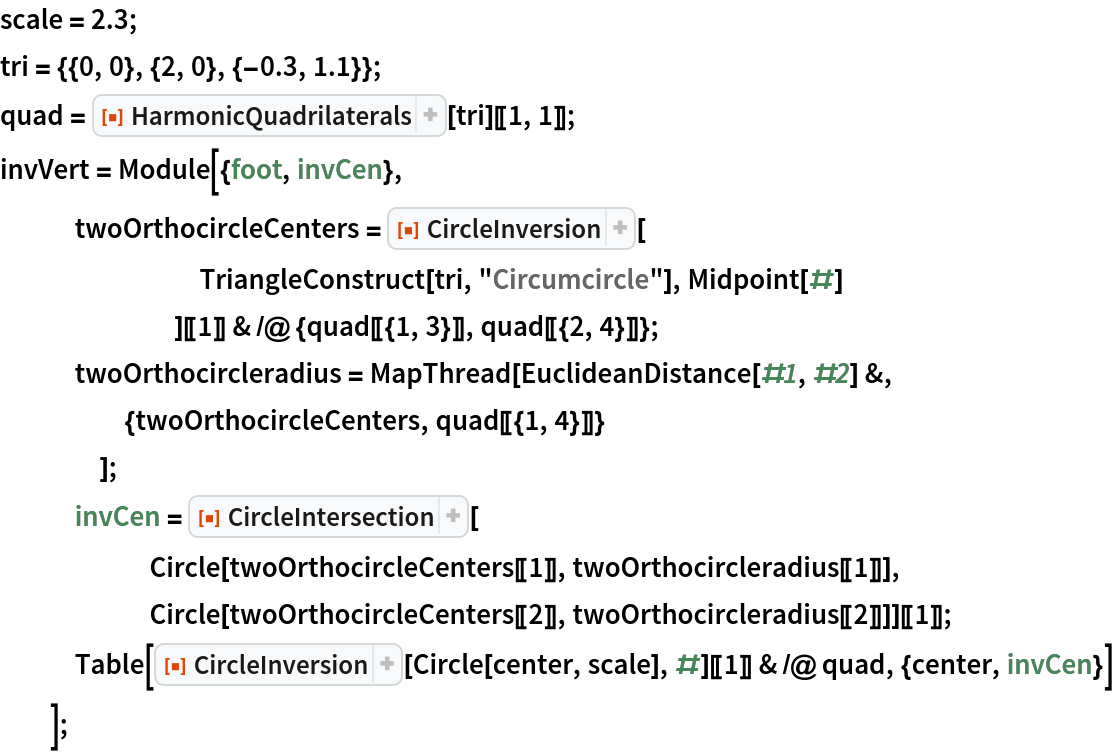The intersections of the two solid orthogonal circles are the proper inversion centers:

 In:=Out=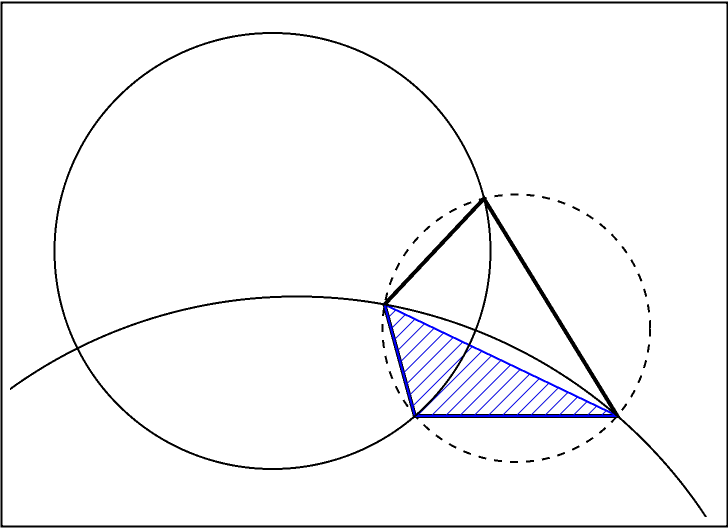Either one can be chosen as the inversion center. The gray hatch-filled polygon is a square for each diagram:

 In:=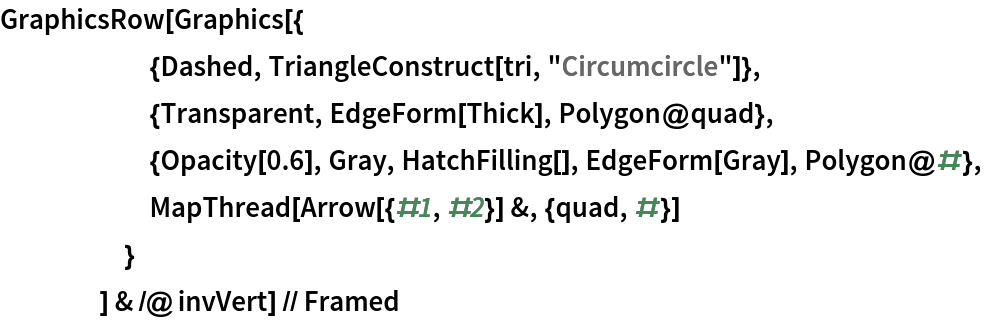Out=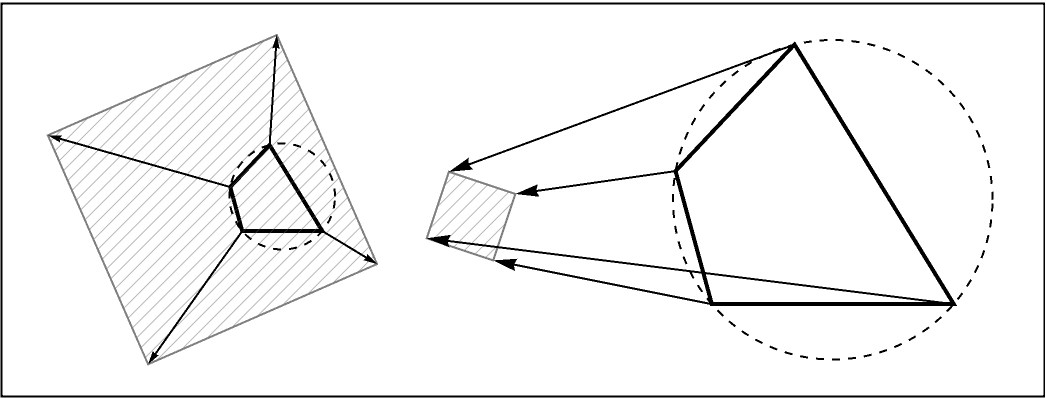A weaker version of the result is that any cyclic quadrilateral (not necessary harmonic) is the inversion of some rectangle. The inversion center is found in the similar way as described above.

Generate a set of random harmonic quadrilaterals:

 In:=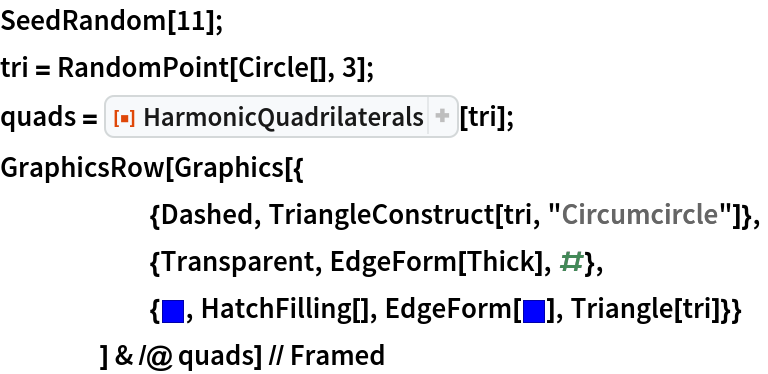Out=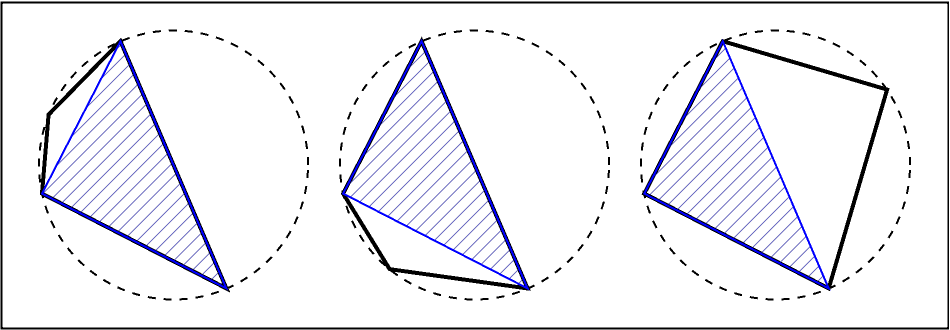Check the distribution of the area of harmonic quadrilaterals in the unit circle:

 In:=Out=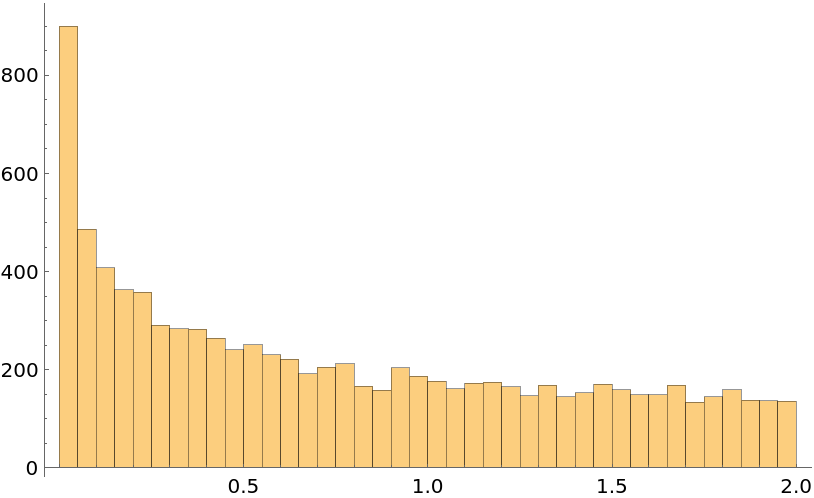Shenghui Yang

## Version History

• 1.0.1 – 04 May 2022
• 1.0.0 – 25 April 2022